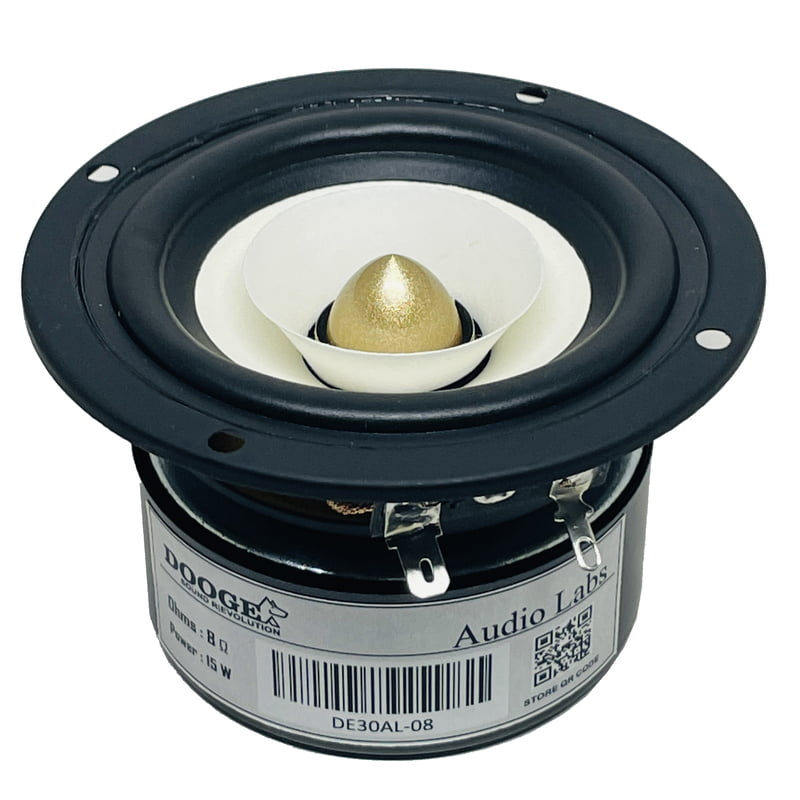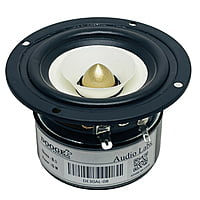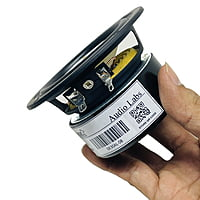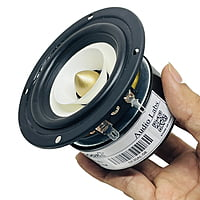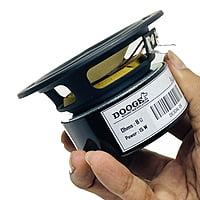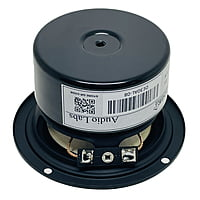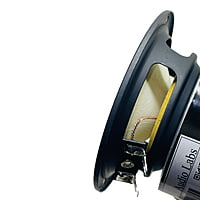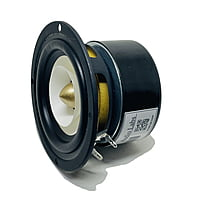# DE30AL-08 Woofer 3" 8Ohms 15 Watts (1 Pcs)

Rs.999.00 Rs.1,799.00
3 inch full range speaker coaxial horn unit aluminum bullet 2 layer paper cone home theater satellite

Impedance:8Ohm

Rated Power:15W

Power range:15-25W

frequency response:80Hz-20KHz

sensitivity:87dB

voice coil is 20.4

f(s) = 157 Hz

Q(ts) = 1.471

V(as) = 0.477 liters (0.01685 cubic feet)

SPL = 81.78 dB SPL 1W/1m

R(e) = 6.976 Ohms

Q(es) = 1.895

SPL = 82.37 dB SPL 2.83 Vrms

C(ms) = 0.399 mm/N

L(e) = 0.1241 mH at 10kHz

Q(ms) = 6.563

BL = 3.059

n(0) = 0.0928 %

M(ms) = 2.579 grams